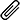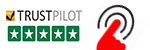High Quality, Fast Delivery, Plagiarism Free - Just in 3 Steps

## Upload Questions Details and Instructions:Select Assignment Files

## Get Assignment Help by Experts

### Plagiarism-Free, Every Time

All of our assignment solution delivers with a dedicated plagiarism report

### Excellent Subject Matter Expert

Get Supreme Assignment Help by highly Skilled Writers

### 100% Satisfaction Guaranteed

Score Higher or Your Money Back# ENG3104 Engineering Simulations and Computations Assignment Help

1       (worth 60 marks)

1.1      Introduction

A cantilever beam is loaded by a uniformly-distributed load (UDL)  (Fig. 1).  Due to the applied force, the beam deforms (is no longer perfectly straight), but the deformation is con- sidered to be so small relative to the length of the beam that there is no horizontal movement (although there is rotation).   The deflection of the beam (the vertical distance between the original location of the centroid and the deformed location of the centroid) at any point along the beam can be calculated  using:Here v is the deflection (up is defined to be positive), w is the unifo rmly-distributed load (force per unit  length) (down is defined to be positive), x is the distance from the  wall, E  is the Young’s modulus for the beam material, I is the second-moment of area for the beam shape and L is the beam’s total length. For this problem, the values of the parameters are:

 w = 3 kN/m E = 200 GPa I L == 986.0 × 106 mm410.5 m

1.2      Requirements

For this assessment item, you must:

1. Solve Eq. (1) using hand calculations to determine the values of v at x = 0, x = L/2 and x = L
1. Solve Eq. (1) using MATLAB to determine the values of v at x = 0, x = L/2 and x = L.

This can be done using the Command Window with screenshots of the work, or using a code written in the MATLAB editor that outputs the results to the Command Window.

You must also produce MATLAB code which:

1. Calculates the value of v from one end of the beam to the other end at 1 mm intervals.
1. Determines the values of v at x = 0, x = L/2 and x = L from the calculation of Requirement 3 and reports the results to the Command Window. The calculations of v from Requirement 2 must be completely independent of these calculations (i.e. should not use any of the same functions).
1. Compares the two different MATLAB methods (Requirements 2 and 4) of calculating v to the hand calculations (Requirement 1) to determine the accuracy of the MATLAB calculations (verify the MATLAB calculations).
1. Reports to the Command Window the outcome of the comparison.
1. Plots the unreformed shape of the beam along with the deformed shape of the beam on the same image. Also plots the deformations at x = 0, x = L/2 and x = L on the same image.
1. Outputs to an ASCII file the distance along the beam and the corresponding deflection as two columns. The perfect file would contain sufficient information for someone to know exactly what the numbers represent.

1.3    Assessment Criteria

Your submission will be assessed using the following scheme.  Note that you are marked based on how well you perform for each category, so the correct answer determined in a basic way will receive half marks and the correct answer determined using an excellent method/code will receive full marks.

 Quality of hand calculations 5 marks Quality of basic MATLAB calculations (Requirement 2) 5 marks Quality of header(s) and comments 5 marks Quality of v calculation at many locations 10 marks Quality of completing Requirement 4 5 marks Quality of verification based on hand calculations 5 marks Quality of plot 10 marks Quality of file output 10 marks Quality of the code 5 marks

2       (worth 35 marks)

2.1      Introduction

Repeat Question 1, but the cantilever beam is loaded by a point load at some distance along the beam. The deflection of the beam at any point along the beam can be calculated using: (2)

where v is the deflection (up is defined to be positive), P is the point load (force) (down is defined to be positive), x is the distance from the wall, E is the Young’s modulus for the beam material, I is the second-moment of area for the beam shape, a is the distance from the wall to the point load and L is the beam’s total length. For this problem, the values of the parameters are:

 P = 7 ken E = 180 GPa Ia == 554.0 × 106 mm413.4 m L = 16.5 m

2.2      Requirements

For this assessment item, you must:

1. Solve Eq. (2) using hand calculations to determine the values of v at x = 0, x = L/2 and x = L.
1. Solve Eq. (2) using MATLAB to determine the values of v at x = 0, x = L/2 and x = L.

This can be done using the Command Window with screenshots of the work or using a code written in the MATLAB editor that outputs the results to the Command Window.

You must also produce MATLAB code which:

1. Verifies the compatibility of the two formulae in Eq. (2) by confirming that v is continuous at a [i.e. both formulae yield the same value of v(x = a)].
1. Calculates the value of v from one end of the beam to the other end at 1 mm intervals.
1. Determines the values of v at x = 0, x = L/2 and x = L from the calculation of Re- quirement 4 and reports the results to the Command Window. The calculations of v from Requirement 2 must be completely independent of these calculations (i.e. should not use any of the same functions).
1. Compares the two different MATLAB methods (Requirements 2 and 5) of calculating v to the hand calculations (Requirement 1) to determine the accuracy of the MATLAB calculations (verify the MATLAB calculations).
1. Reports to the Command Window the outcome of the comparison.
1. Plots the undeformed shape of the beam along with the deformed shape of the beam on the same image. Also plots the deformations at x = 0, x = L/2 and x = L on the same image.

2.3    Assessment Criteria

Your submission will be assessed using the following scheme.  Note that you are marked based on how well you perform for each category, so the correct answer determined in a basic way will receive half marks and the correct answer determined using an excellent method/code will receive full marks.

 Quality of hand calculations 5 marks Quality of header(s) and comments 5 marks Quality of MATLAB calculations 10 marks Quality of verifications 5 marks Quality of the code 10 marks

3       (worth 5 marks)

You are to write a brief report (about 100 words, excluding any code), which includes:

1. A description of an instance during the writing of your code for this assignment where there was a problem (e.g. a bug, an error, an unexpected result) or the most challenging aspect to overcome.
1. What steps you took to overcome the problem or challenge (including any code you wrote to test the problem/challenge).
1. The code before you resolved the problem or challenge, highlighting the line(s) of code where the problem or challenge occurred.
1. The code after you resolved the problem/overcame the challenge.
1. Code from the MATLAB editor is to be copied into Word; screenshots should be taken of the Command window.The problem report will be assessed using the following scheme:
 Description Marks Excellent description of a difficult problem and effective solution 5 Good description of a moderate problem and useful solution 4 Reasonable description of a genuine problem with a pragmatic solution 3 Poor description or Problem is not very challenging or Solution is not effective/efficient 2 Problem is trivial  or Solution is poor 1 No report or description does not include a genuine problem 0

Submission

Submit your code, with the output file that is produced in Question 1, by the due date to the Study Desk. Submit your hand calculations as a pdf file (this file does NOT require selectable text:  it merely needs to be able to be opened). Submit your problem report as a pdf file that contains selectable text, i.e. you can use a mouse cursor to highlight text which you can copy and paste (your assignment will not be marked if the problem report pdf file does not satisfy this requirement).

Chat with our 24x 7 Online Agents CLICK CHAT NOW

Check out our Engineering Assignment Help Services

CDR For Australia | Sample CDR for Australian Migration | CDR Sample Writing Services

#### Get This Answer for Study Help

If you need study assistance with writing your questions and answers, our professional assignment writing service is here to help!

#### Content Removal Request

If you are the original writer or copyright-authorized owner of this article and no longer wish to have, your work published on casestudyhelp.com, then please Request for removal of this content.

Top